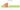# MTn

## Mozambican Metical

Mozambique's currency, the metical, is denoted by the letters MZN or MT. The nominal unit of currency is the centavo. The word "metical" is derived from the Arabic word "mthql," which also serves as a weight unit and a different term for the gold dinar coin that was used in much of Africa up until the 19th century.

#### how has the value of the currency changed in the last year?## USD 1 = MZN 63.875

How much is10 US dollars worth inMozambican meticals?
At the current exchange rate, 10 US dollars is worth 638.75 Mozambican meticals
How much is50 US dollars worth inMozambican meticals?
At the current exchange rate, 50 US dollars is worth 3,193.75 Mozambican meticals
How much is100 US dollars worth inMozambican meticals?
At the current exchange rate, 100 US dollars is worth 6,387.5 Mozambican meticals
How much is500 US dollars worth inMozambican meticals?
At the current exchange rate, 500 US dollars is worth 31,937.5 Mozambican meticals
How much is2,000 US dollars worth inMozambican meticals?
At the current exchange rate, 2,000 US dollars is worth 127,749.98 Mozambican meticals
How much is10 US dollars worth inMozambican meticals?
At the current exchange rate, 10 US dollars is worth 638.75 Mozambican meticals
How much is50 US dollars worth inMozambican meticals?
At the current exchange rate, 50 US dollars is worth 3,193.75 Mozambican meticals
How much is100 US dollars worth inMozambican meticals?
At the current exchange rate, 100 US dollars is worth 6,387.5 Mozambican meticals
How much is500 US dollars worth inMozambican meticals?
At the current exchange rate, 500 US dollars is worth 31,937.5 Mozambican meticals
How much is2,000 US dollars worth inMozambican meticals?
At the current exchange rate, 2,000 US dollars is worth 127,749.98 Mozambican meticals